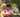### Find Missing Element

January 16, 2020

Find Missing Element

An array A consisting of N different integers is given. The array contains integers in the range [1..(N + 1)], which means that exactly one element is missing. Your goal is to find that missing element.

Write a function:
function solution(A);
that, given an array A, returns the value of the missing element.
For example, given array A such that:
A = 2
A = 3
A = 1
A = 5
the function should return 4, as it is the missing element.
Write an efficient algorithm for the following assumptions:
• N is an integer within the range [0..100,000];
• the elements of A are all distinct;
• each element of array A is an integer within the range [1..(N + 1)].

Use trapezoid area (calculated with height=A.length+1) minus existing array's summation to get the missing element.

``````function missingElement(A) {
console.log(A)
var length = A.length;

const height = length + 1
const sum = (1+ height) * height /2 // trapezoid area
var sumMinusMissing = 0;
for (i = 0; i < length; i++) {
sumMinusMissing += A[i];
}
return sum - sumMinusMissing;
}
A1 = [2, 3, 1, 5]
console.log("missingElement : ", missingElement(A1))``````Posted by Tai Lu ( 呂台生 ) who's building useful things.You should follow him on Twitter